# Mean State

Period Mean (original grids) [K]
Model Period Mean (intersection) [K]
Model Period Mean (complement) [K]
Benchmark Period Mean (intersection) [K]
Benchmark Period Mean (complement) [K]
Bias [K]
RMSE [K]
Phase Shift [months]
Bias Score 
RMSE Score 
Seasonal Cycle Score 
Spatial Distribution Score 
Interannual Variability Score 
Overall Score 
Benchmark [-] 293.
CRUNCEPv7 [-] 295. 295. 298. 293. 296. 2.08 2.28 0.884 0.196 0.502 0.893 0.999 0.799 0.648
GSWP3v1 [-] 293. 293. 297. 293. 296. 0.833 1.28 0.623 0.462 0.492 0.928 0.998 0.767 0.690
Period Mean (original grids) [K]
Model Period Mean (intersection) [K]
Model Period Mean (complement) [K]
Benchmark Period Mean (intersection) [K]
Benchmark Period Mean (complement) [K]
Bias [K]
RMSE [K]
Phase Shift [months]
Bias Score 
RMSE Score 
Seasonal Cycle Score 
Spatial Distribution Score 
Interannual Variability Score 
Overall Score 
Benchmark [-] 293.
CRUNCEPv7 [-] 295. 295. 297. 293. 294. 1.87 2.50 0.334 0.503 0.633 0.968 0.959 0.889 0.764
GSWP3v1 [-] 294. 294. 296. 293. 294. 1.08 1.45 0.183 0.641 0.746 0.984 0.985 0.859 0.827
Period Mean (original grids) [K]
Model Period Mean (intersection) [K]
Model Period Mean (complement) [K]
Benchmark Period Mean (intersection) [K]
Benchmark Period Mean (complement) [K]
Bias [K]
RMSE [K]
Phase Shift [months]
Bias Score 
RMSE Score 
Seasonal Cycle Score 
Spatial Distribution Score 
Interannual Variability Score 
Overall Score 
Benchmark [-] 288.
CRUNCEPv7 [-] 291. 291. 294. 288. 293. 2.46 2.65 0.692 0.448 0.747 0.915 0.999 0.827 0.780
GSWP3v1 [-] 289. 289. 293. 288. 293. 0.967 1.33 0.517 0.673 0.786 0.938 1.00 0.843 0.838
Period Mean (original grids) [K]
Model Period Mean (intersection) [K]
Model Period Mean (complement) [K]
Benchmark Period Mean (intersection) [K]
Benchmark Period Mean (complement) [K]
Bias [K]
RMSE [K]
Phase Shift [months]
Bias Score 
RMSE Score 
Seasonal Cycle Score 
Spatial Distribution Score 
Interannual Variability Score 
Overall Score 
Benchmark [-] 256.
CRUNCEPv7 [-] 257. 257. 261. 256. 261. 0.683 1.93 0.0676 0.931 0.907 0.995 0.998 0.886 0.937
GSWP3v1 [-] 256. 256. 264. 256. 261. 0.444 1.75 0.0394 0.932 0.918 0.997 0.999 0.908 0.945
Period Mean (original grids) [K]
Model Period Mean (intersection) [K]
Model Period Mean (complement) [K]
Benchmark Period Mean (intersection) [K]
Benchmark Period Mean (complement) [K]
Bias [K]
RMSE [K]
Phase Shift [months]
Bias Score 
RMSE Score 
Seasonal Cycle Score 
Spatial Distribution Score 
Interannual Variability Score 
Overall Score 
Benchmark [-] 294.
CRUNCEPv7 [-] 297. 297. 298. 294. 295. 3.14 3.28 1.65 0.0594 0.481 0.746 0.921 0.743 0.572
GSWP3v1 [-] 295. 295. 297. 294. 295. 1.18 1.64 1.26 0.294 0.504 0.813 0.958 0.820 0.649
Period Mean (original grids) [K]
Model Period Mean (intersection) [K]
Model Period Mean (complement) [K]
Benchmark Period Mean (intersection) [K]
Benchmark Period Mean (complement) [K]
Bias [K]
RMSE [K]
Phase Shift [months]
Bias Score 
RMSE Score 
Seasonal Cycle Score 
Spatial Distribution Score 
Interannual Variability Score 
Overall Score 
Benchmark [-] 265.
CRUNCEPv7 [-] 266. 266. 272. 265. 269. 0.573 1.46 0.0284 0.937 0.931 0.998 0.993 0.971 0.960
GSWP3v1 [-] 265. 265. 272. 265. 269. -0.203 1.13 0.0201 0.946 0.944 0.999 0.998 0.964 0.966
Period Mean (original grids) [K]
Model Period Mean (intersection) [K]
Model Period Mean (complement) [K]
Benchmark Period Mean (intersection) [K]
Benchmark Period Mean (complement) [K]
Bias [K]
RMSE [K]
Phase Shift [months]
Bias Score 
RMSE Score 
Seasonal Cycle Score 
Spatial Distribution Score 
Interannual Variability Score 
Overall Score 
Benchmark [-] 274.
CRUNCEPv7 [-] 275. 275. 285. 274. 280. 1.34 2.23 0.0239 0.841 0.887 0.998 0.998 0.924 0.923
GSWP3v1 [-] 275. 274. 285. 274. 280. 0.788 1.57 0.0129 0.876 0.925 0.999 0.999 0.927 0.942
Period Mean (original grids) [K]
Model Period Mean (intersection) [K]
Model Period Mean (complement) [K]
Benchmark Period Mean (intersection) [K]
Benchmark Period Mean (complement) [K]
Bias [K]
RMSE [K]
Phase Shift [months]
Bias Score 
RMSE Score 
Seasonal Cycle Score 
Spatial Distribution Score 
Interannual Variability Score 
Overall Score 
Benchmark [-] 291.
CRUNCEPv7 [-] 292. 292. 298. 291. 296. 1.15 2.14 0.321 0.630 0.795 0.975 0.991 0.858 0.841
GSWP3v1 [-] 291. 291. 297. 291. 296. 0.700 1.49 0.227 0.721 0.846 0.981 0.999 0.928 0.887
Period Mean (original grids) [K]
Model Period Mean (intersection) [K]
Model Period Mean (complement) [K]
Benchmark Period Mean (intersection) [K]
Benchmark Period Mean (complement) [K]
Bias [K]
RMSE [K]
Phase Shift [months]
Bias Score 
RMSE Score 
Seasonal Cycle Score 
Spatial Distribution Score 
Interannual Variability Score 
Overall Score 
Benchmark [-] 259.
CRUNCEPv7 [-] 259. 259. 261. 259. 260. -0.000922 1.58 0.0247 0.961 0.911 0.998 0.999 0.911 0.949
GSWP3v1 [-] 259. 259. 263. 259. 260. -0.185 1.46 0.0250 0.956 0.923 0.998 1.00 0.920 0.953
Period Mean (original grids) [K]
Model Period Mean (intersection) [K]
Model Period Mean (complement) [K]
Benchmark Period Mean (intersection) [K]
Benchmark Period Mean (complement) [K]
Bias [K]
RMSE [K]
Phase Shift [months]
Bias Score 
RMSE Score 
Seasonal Cycle Score 
Spatial Distribution Score 
Interannual Variability Score 
Overall Score 
Benchmark [-] 287.
CRUNCEPv7 [-] 290. 290. 291. 287. 289. 2.63 2.78 0.197 0.578 0.838 0.986 0.995 0.907 0.857
GSWP3v1 [-] 289. 289. 290. 287. 289. 1.23 1.39 0.123 0.761 0.893 0.992 1.00 0.945 0.914
Period Mean (original grids) [K]
Model Period Mean (intersection) [K]
Model Period Mean (complement) [K]
Benchmark Period Mean (intersection) [K]
Benchmark Period Mean (complement) [K]
Bias [K]
RMSE [K]
Phase Shift [months]
Bias Score 
RMSE Score 
Seasonal Cycle Score 
Spatial Distribution Score 
Interannual Variability Score 
Overall Score 
Benchmark [-] 277.
CRUNCEPv7 [-] 278. 278. 281. 277. 281. 0.964 1.59 0.125 0.806 0.874 0.992 0.993 0.921 0.910
GSWP3v1 [-] 277. 277. 280. 277. 281. 0.142 1.12 0.120 0.861 0.908 0.992 0.994 0.943 0.934
Period Mean (original grids) [K]
Model Period Mean (intersection) [K]
Model Period Mean (complement) [K]
Benchmark Period Mean (intersection) [K]
Benchmark Period Mean (complement) [K]
Bias [K]
RMSE [K]
Phase Shift [months]
Bias Score 
RMSE Score 
Seasonal Cycle Score 
Spatial Distribution Score 
Interannual Variability Score 
Overall Score 
Benchmark [-] 281.
CRUNCEPv7 [-] 277. 282. 241. 280. 287. 1.51 2.27 0.380 0.654 0.797 0.952 0.999 0.896 0.849
GSWP3v1 [-] 277. 281. 244. 280. 287. 0.726 1.47 0.250 0.760 0.835 0.970 0.999 0.909 0.885
Period Mean (original grids) [K]
Model Period Mean (intersection) [K]
Model Period Mean (complement) [K]
Benchmark Period Mean (intersection) [K]
Benchmark Period Mean (complement) [K]
Bias [K]
RMSE [K]
Phase Shift [months]
Bias Score 
RMSE Score 
Seasonal Cycle Score 
Spatial Distribution Score 
Interannual Variability Score 
Overall Score 
Benchmark [-] 289.
CRUNCEPv7 [-] 289. 289. 292. 288. 292. 0.626 1.90 0.298 0.789 0.838 0.977 0.995 0.909 0.891
GSWP3v1 [-] 289. 289. 292. 288. 292. 0.668 1.30 0.222 0.850 0.883 0.983 1.00 0.909 0.918
Period Mean (original grids) [K]
Model Period Mean (intersection) [K]
Model Period Mean (complement) [K]
Benchmark Period Mean (intersection) [K]
Benchmark Period Mean (complement) [K]
Bias [K]
RMSE [K]
Phase Shift [months]
Bias Score 
RMSE Score 
Seasonal Cycle Score 
Spatial Distribution Score 
Interannual Variability Score 
Overall Score 
Benchmark [-] 260.
CRUNCEPv7 [-] 260. 260. 259. 260. 262. 0.273 1.66 0.0664 0.935 0.905 0.996 0.983 0.896 0.937
GSWP3v1 [-] 260. 260. 262. 260. 262. 0.473 1.54 0.0471 0.937 0.914 0.997 0.997 0.920 0.946
Period Mean (original grids) [K]
Model Period Mean (intersection) [K]
Model Period Mean (complement) [K]
Benchmark Period Mean (intersection) [K]
Benchmark Period Mean (complement) [K]
Bias [K]
RMSE [K]
Phase Shift [months]
Bias Score 
RMSE Score 
Seasonal Cycle Score 
Spatial Distribution Score 
Interannual Variability Score 
Overall Score 
Benchmark [-] 289.
CRUNCEPv7 [-] 291. 291. 295. 289. 293. 2.99 3.43 0.543 0.380 0.707 0.947 0.992 0.872 0.768
GSWP3v1 [-] 289. 289. 294. 289. 293. 1.38 2.00 0.342 0.569 0.766 0.965 0.999 0.902 0.828
Period Mean (original grids) [K]
Model Period Mean (intersection) [K]
Model Period Mean (complement) [K]
Benchmark Period Mean (intersection) [K]
Benchmark Period Mean (complement) [K]
Bias [K]
RMSE [K]
Phase Shift [months]
Bias Score 
RMSE Score 
Seasonal Cycle Score 
Spatial Distribution Score 
Interannual Variability Score 
Overall Score 
Benchmark [-] 280.
CRUNCEPv7 [-] 282. 282. 291. 280. 287. 2.04 2.23 0.0503 0.782 0.912 0.997 0.993 0.948 0.924
GSWP3v1 [-] 281. 281. 290. 280. 287. 1.10 1.32 0.0253 0.872 0.934 0.998 1.00 0.971 0.951
Period Mean (original grids) [K]
Model Period Mean (intersection) [K]
Model Period Mean (complement) [K]
Benchmark Period Mean (intersection) [K]
Benchmark Period Mean (complement) [K]
Bias [K]
RMSE [K]
Phase Shift [months]
Bias Score 
RMSE Score 
Seasonal Cycle Score 
Spatial Distribution Score 
Interannual Variability Score 
Overall Score 
Benchmark [-] 268.
CRUNCEPv7 [-] 269. 269. 274. 268. 274. 1.09 1.64 0.0359 0.885 0.908 0.998 0.997 0.946 0.940
GSWP3v1 [-] 269. 269. 274. 268. 274. 0.585 1.21 0.0363 0.918 0.922 0.998 0.998 0.948 0.951
Period Mean (original grids) [K]
Model Period Mean (intersection) [K]
Model Period Mean (complement) [K]
Benchmark Period Mean (intersection) [K]
Benchmark Period Mean (complement) [K]
Bias [K]
RMSE [K]
Phase Shift [months]
Bias Score 
RMSE Score 
Seasonal Cycle Score 
Spatial Distribution Score 
Interannual Variability Score 
Overall Score 
Benchmark [-] 276.
CRUNCEPv7 [-] 277. 277. 283. 276. 280. 1.82 2.53 0.0322 0.758 0.838 0.997 0.999 0.897 0.888
GSWP3v1 [-] 277. 277. 283. 276. 280. 1.58 2.03 0.0332 0.783 0.892 0.998 0.998 0.941 0.917
Period Mean (original grids) [K]
Model Period Mean (intersection) [K]
Model Period Mean (complement) [K]
Benchmark Period Mean (intersection) [K]
Benchmark Period Mean (complement) [K]
Bias [K]
RMSE [K]
Phase Shift [months]
Bias Score 
RMSE Score 
Seasonal Cycle Score 
Spatial Distribution Score 
Interannual Variability Score 
Overall Score 
Benchmark [-] 294.
CRUNCEPv7 [-] 296. 296. 298. 294. 294. 2.24 2.51 1.97 0.138 0.530 0.689 1.00 0.881 0.628
GSWP3v1 [-] 294. 294. 297. 294. 294. 0.878 1.34 1.08 0.417 0.551 0.837 0.994 0.877 0.705
Period Mean (original grids) [K]
Model Period Mean (intersection) [K]
Model Period Mean (complement) [K]
Benchmark Period Mean (intersection) [K]
Benchmark Period Mean (complement) [K]
Bias [K]
RMSE [K]
Phase Shift [months]
Bias Score 
RMSE Score 
Seasonal Cycle Score 
Spatial Distribution Score 
Interannual Variability Score 
Overall Score 
Benchmark [-] 290.
CRUNCEPv7 [-] 292. 292. 294. 290. 288. 2.56 2.70 0.678 0.390 0.750 0.907 0.999 0.922 0.786
GSWP3v1 [-] 291. 291. 293. 290. 288. 1.13 1.35 0.470 0.631 0.800 0.937 1.00 0.936 0.851
Period Mean (original grids) [K]
Model Period Mean (intersection) [K]
Model Period Mean (complement) [K]
Benchmark Period Mean (intersection) [K]
Benchmark Period Mean (complement) [K]
Bias [K]
RMSE [K]
Phase Shift [months]
Bias Score 
RMSE Score 
Seasonal Cycle Score 
Spatial Distribution Score 
Interannual Variability Score 
Overall Score 
Benchmark [-] 278.
CRUNCEPv7 [-] 280. 280. 285. 278. 281. 2.73 3.31 0.255 0.444 0.750 0.972 1.00 0.921 0.806
GSWP3v1 [-] 279. 279. 284. 278. 281. 1.40 2.27 0.106 0.580 0.795 0.990 1.00 0.921 0.847

# Temporally integrated period mean

BENCHMARK MEAN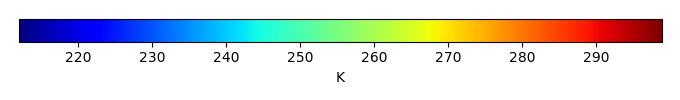MODEL MEANBIAS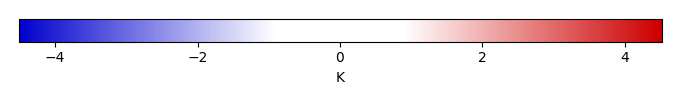BIAS SCORERMSE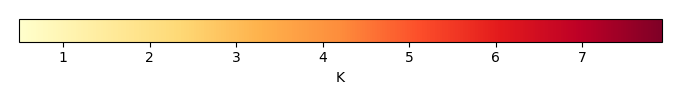RMSE SCOREBENCHMARK INTERANNUAL VARIABILITY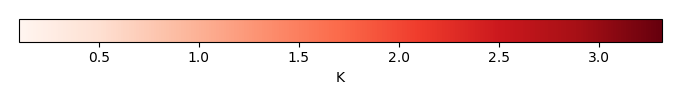MODEL INTERANNUAL VARIABILITYINTERANNUAL VARIABILITY SCOREBENCHMARK MAX MONTHMODEL MAX MONTHDIFFERENCE IN MAX MONTH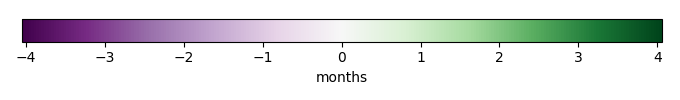SEASONAL CYCLE SCORESPATIAL TAYLOR DIAGRAMMODEL COLORS# Spatially integrated regional mean

MODEL COLORSREGIONAL MEANANNUAL CYCLEMONTHLY ANOMALYANNUAL CYCLE# All Models

BenchmarkCRUNCEPv7GSWP3v1# Data Information

creation_date: Fri Feb 8 14:17:30 PST 2019

history: 2018-01-15: download from https://crudata.uea.ac.uk/cru/data/hrg/cru_ts_4.01/cruts.1709081022.v4.01/ 2019-02-06: converted and created to netCDF4 for ILAMB package

original_resolution: 0.5x0.5

resolution: 0.5x0.5

original_frequency: mon

frequency: mon

references: Harris et al., 2014, Int. J. Climatol., doi:10.1002/joc.3711

institution: Original Data held at British Atmospheric Data Centre, RAL, UK.

source: Run ID = 1709081022. Data generated from:TMN derived from gridded TMP & DTR absolutes, DTR in turn derived from tmn.1708171135.dtb, tmx.1708171215.dtb

title: CRU TS4.01 Minimum Temperature

Conventions: CF-1.7